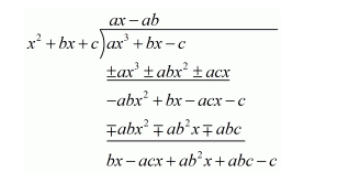# If the polynomial f(x) = ax3 + bx − c is divisible

Question:

If the polynomial $l(x)=a x^{3}+b x-c$ is divisible by the polynomial $g(x)=x^{2}+b x+c$, then $a b=$

(a) 1

(b) $\frac{1}{c}$

(c) $-1$

(d) $-\frac{1}{c}$

Solution:

We have to find the value of $a b$

Given $f(x)=a x^{3}+b x-c$ is divisible by the polynomial $g(x)=x^{2}+b x+c$We must have

$b x-a c x+a b^{2} x+a b c-c=0$, for all $x$

So put $x=0$ in this equation

$x\left(b-a c+a b^{2}\right)+c(a b-1)=0$

$c(a b-1)=0$

Since $c \neq 0$, so

$a b-1=0$

$\Rightarrow a b=1$

Hence, the correct alternative is  (a)